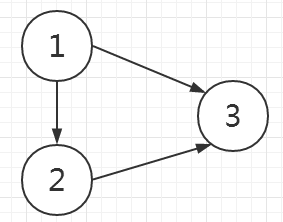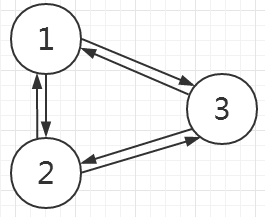# 洛谷 P2783 有机化学之神偶尔会做作弊 题解【tarjan】【最大环】

话说为什么要考信息竞赛生的化学……

## 题目背景

XS中学化学竞赛组教练是一个酷爱炉石的人。

“第1354题怎么做”<–手语 他问道。

## 题目描述3 2
1 2
2 3
2
1 2
2 3

10
10

## 说明

1<n<=10000,1<m<=50000

## （两个碳不成环）

题目大意是把所有环缩成一个点，最后求无环图（树）上两点间距离。

缩点，本该是有向图中的概念，但是这里缩环为点的概念，其实是“换汤不换药”。无向图的环和有向图的本质是一样的，我们可以通过tarjan算法来完成这个任务。

对于有向图，如下图所示的就不能成环，而无向图就可以：它们之间的区别除了双向边以外，一点区别都没有，因此我们可以直接和缩点洛谷P3387缩点一样做。两个题所不同的是，连接在环上的边如果分别连接到环外，那么缩点后的点要添加上这条边。

需要注意一点，缩点是可以滞后进行的，也就是说，dfs过程中只需要存储这个点缩点后变成了哪个点，缩点操作是在dfs后统一进行的。就算缩点被更新了，我们只需要最后再统计一遍就可以了。

缩点这一过程是通过del数组来存储的，del数组更像是一个并查集，例如del=2,5号被缩进了2号，但最后del=1,2号缩进了1号。那么5号和2号都是被缩进1号的，我们最后要把5号和2号连接在环外的点全部连在1号上，这样就构建出来了最终的树。

我们在最终的树上求lca就能把树上各两点距离+1求出来了。如果询问中两个点被缩到一起，是不会执行dfs的，需要特判。同时ans不能被更新，要保持第一次被查询时的值（不然状态改变求出的就不是真正的lca了）。

## Code（bfs1）：

#include<cstdio>
#include<cstring>
#include<cstdlib>
struct node
{
int n,cnt;
node *nxt,*from;
node(int n)
{
this->n=n;
cnt=0;
nxt=NULL;
from=NULL;
}
node(int n,int cnt,node *from)
{
this->n=n;
this->cnt=cnt;
nxt=NULL;
this->from=from;
}
node(){nxt=NULL;from=NULL;}
};
int dfn,cnt=0;
int n,m;
int S,top=0;
int del,pre;
int dfind(int x)
{
if(del[x]==x)
return x;
return del[x]=dfind(del[x]);
}
void dfs(int x)//这里用的是栈模拟dfs，效率略有提升，不过不太直观
{
S[++top]=x;//将第一个点进栈
S=0;//第一个点没有父亲结点
while(top)
{
x=S[top];
if(!dfn[x])//需要时间戳
dfn[x]=++cnt;
while(p->nxt!=NULL)
{
p=p->nxt;
if(p->n==S[top-1])//保证不是父亲（父亲一定是它栈下面的一个数）
continue;
if(!dfn[p->n])
{
S[++top]=p->n;
pre[p->n]=x;//pre也用作存父亲
top++;//默认会弹出一下，为保证不弹出，我们将top++
break;
}
if(dfn[p->n]<dfn[x]&&p->n!=pre[x])
//说明找到环了
{
int t=top;
while(S[t]!=p->n&&t)
{
del[S[t]]=dfind(p->n);//删点时类似并查集的操作
t--;
}
}
}
top--;
}
}
int dpt,ans;
bool vis;
int fa;
int my_find(int x)//tarjan所用的并查集
{
if(fa[x]==x)
return x;
return fa[x]=my_find(fa[x]);
}
int cal(int x,int y,int lca)
{
return dpt[x]+dpt[y]-2*dpt[lca];
}
void tarjan(int x,int from)//求lca更新距离
{
vis[x]=true;
while(p->nxt!=NULL)
{
p=p->nxt;
if(p->n==from)//不能找自己的父亲
continue;
dpt[p->n]=dpt[x]+1;//树上前缀和
//看是否有查询
tarjan(p->n,x);
while(P->nxt!=NULL)
{
P=P->nxt;
if(vis[P->n])
{
if(ans[P->cnt]==-1)//前缀和
ans[P->cnt]=cal(p->n,P->n,my_find(P->n));
P->from->nxt=P->nxt;//剪枝，把这个询问删掉
}
}
}
fa[x]=from;
}
{
char c=getchar();int x=0;
while(c<'0'||c>'9') c=getchar();
while(c>='0'&&c<='9') {x=x*10+c-'0';c=getchar();}
return x;
}
void print(int x)//二进制输出
{
int stk;
int t=0;
while(x)
{
stk[++t]=x&1;
x>>=1;
}
for(int i=t;i>=1;i--)
printf("%d",stk[i]);
printf("\n");
}
int main()
{
int u,v;
for(int i=1;i<=n;i++)
{
del[i]=i;
fa[i]=i;
}
memset(ans,-1,sizeof(ans));
for(int i=1;i<=m;i++)
{
tail[u]->nxt=new node(v);
tail[u]=tail[u]->nxt;
tail[v]->nxt=new node(u);
tail[v]=tail[v]->nxt;
}
memset(dfn,0,sizeof(dfn));
pre=0;
dfs(1);

//重新构图

for(int i=1;i<=n;i++)
{
while(p->nxt!=NULL)
{
p=p->nxt;
if(dfind(p->n)==dfind(i))
continue;
int r=dfind(i);
Tail[r]->nxt=new node(p->n);
Tail[r]=Tail[r]->nxt;

}
}

//tarjan求树上两点距离
int tt;
for(int i=1;i<=tt;i++)
{
u=dfind(u);
v=dfind(v);
if(u==v)
ans[i]=0;
}
dpt=0;
memset(vis,0,sizeof(vis));
tarjan(1,0);//求lca
for(int i=1;i<=tt;i++)
print(ans[i]+1);
return 0;
}


## bfs2：（完全模仿tarjan求强连通分量）注释同上，更多细节参见：tarjan学习笔记缩点

void dfs(int x,int from)
{
dfn[x]=++cnt;
low[x]=dfn[x];
S[++top]=x;
while(p->nxt!=NULL)
{
p=p->nxt;
if(p->n==from)
continue;
if(!dfn[p->n])
{
dfs(p->n,x);
low[x]=min(low[p->n],low[x]);
}
else
low[x]=min(low[x],dfn[p->n]);
}
if(low[x]==dfn[x])
{
while(S[top]!=x)
{
del[S[top]]=dfind(x);
top--;
}
del[S[top]]=dfind(x);//x的子树遍历完了
top--;
}
}

### 2 说点什么0 Followers

Most reacted comment
0 Comment authors
Recent comment authors
SubscribewpDiscuz